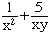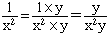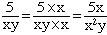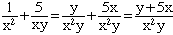Quandaries and Queries Name: Ashley Who is asking: Student Level: Secondary Question: Could you please show steps to thank you so much. (/ means fraction) 1/x(squared) + 5/xy Hi Ashley, You haven't told me what the question is so I will have to guess. I expect the question is to add the two fractions or write the sum with a common denominator.The denominators are x 2 = xx and xy. To construct a common denominator you need xx for the first fraction and xy for the second. The most economical way to do this is to use the denominator xxy = x 2y To change the denominator of the first fraction to  x 2y you need to multiply it by y. Thus you need to also multiply the numerator by y. HenceTo change the denominator of the second fraction to  x 2y you need to multiply it by x. Thus you need to also multiply the numerator by x. HenceFinallyPenny Go to Math Central